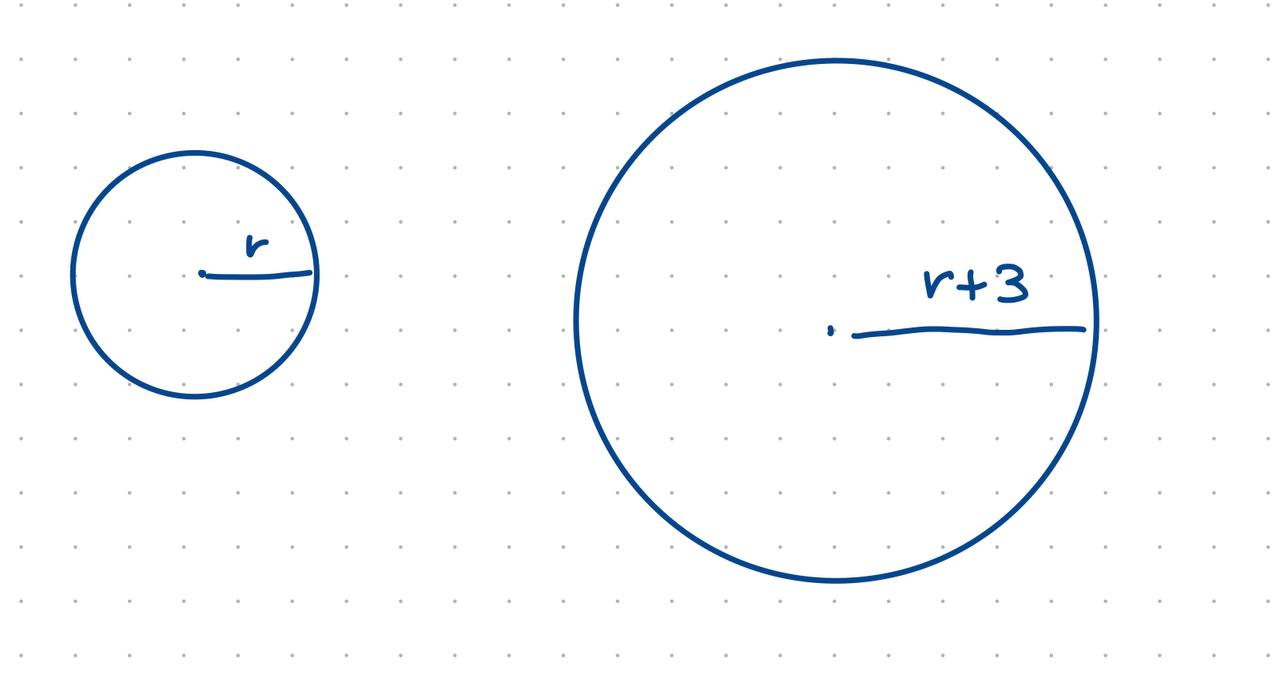# consider two circles, a smaller one and a larger one. If the larger one has a radius that is 3 feet larger than that of the smaller circle and the ratio of the circumferences is 2:1, what are the radii of the two circles?Luciano Webster 2022-07-29 Answered
Consider two circles, a smaller one and a larger one. If the larger one has a radius that is 3 feet larger than that of the smaller circle and the ratio of the circumferences is 2:1, what are the radii of the two circles?
You can still ask an expert for help

• Questions are typically answered in as fast as 30 minutes

Solve your problem for the price of one coffee

• Math expert for every subject
• Pay only if we can solve itnezivande0u
Begin by diagramming the two circles just as it states in theproblemNow determine the circumference of each circle
On the Right, we get 2$\pi$(r+3)
On the Left, we get 2$\pi$r
The ratio of these must be 2:1
$\frac{2}{1}=\frac{2\pi \left(r+3\right)}{2\pi r}=\frac{r+3}{r}$
now we can use the first and last fractions and use crossmultiplication to solve for r
$\left(2\right)\left(r\right)=\left(1\right)\left(r+3\right)\phantom{\rule{0ex}{0ex}}2r+r+3\phantom{\rule{0ex}{0ex}}r=3$
which is the radius of the smaller circle
r+3=6is the radius of the larger circle